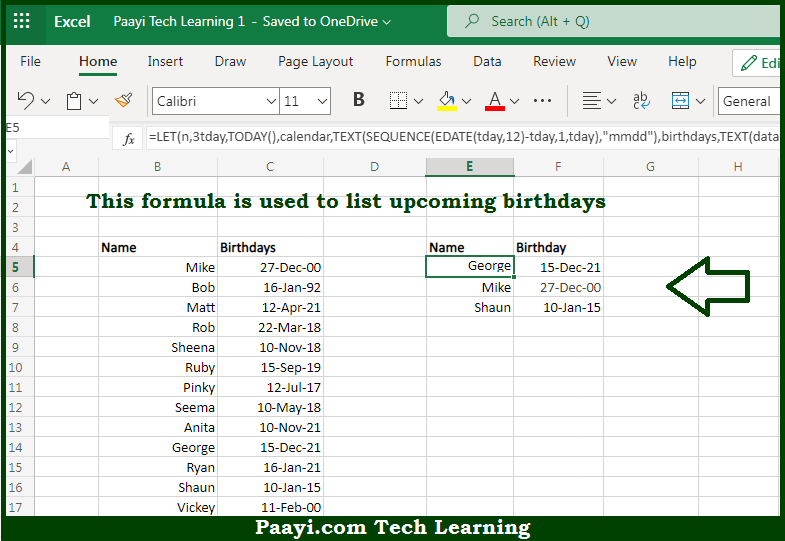# Learn How to List Upcoming Birthdays in Microsoft Excel

Written by | 0 Comments | 705 Views

In this article, you will learn how to evaluate things in Dynamic Arrays with formulas in Microsoft Excel using a single/combination(s) of functions. You will also know How to List Upcoming Birthdays and see the generic formula.

Learn How to List Upcoming Birthdays in Microsoft Excel

The main purpose of this formula is to list the next n upcoming birthdays from a larger table of names and birthdays. Here we will learn how to list upcoming birthdays in the given data range in the workbook in Microsoft Excel. That implies, with the help of a formula based on the INDEX, XMATCH, and SORTBY functions you can able to list the next n upcoming birthdays from a larger table of names and birthdays. So, with the help of this formula, you can able to list upcoming birthdays in the given data range in the workbook in Microsoft Excel.

General Formula to List Upcoming Birthdays

=LET(n,7,tday,TODAY(),calendar,TEXT(SEQUENCE(EDATE(tday,12)-tday,1,tday),"mmdd"),

birthdays,TEXT(data[Birthday],"mmdd"),sorted,SORTBY(data,XMATCH(birthdays,calendar)),

INDEX(sorted,SEQUENCE(MIN(n,ROWS(data))),SEQUENCE(1,COLUMNS(data))))

The Explanation for Listing Upcoming BirthdaysSo we know that with the help of the given formula above you can able to list the next n upcoming birthdays from a larger table of names and birthdays. Here we will learn how to list upcoming birthdays in the given data range in the workbook in Microsoft Excel. As we know that the main challenge here is to sort the list of birthdays into a rolling list of upcoming dates.

Here the formula functions in two parts:

• Sort all birthdays according to a rolling calendar and,
• Return the first 7 birthdays from the sorted list.

So, with the help of this formula, you can able to list the next n upcoming birthdays from a larger table of names and birthdays. So now you have learned how to list upcoming birthdays in the given data range in the workbook in Microsoft Excel.